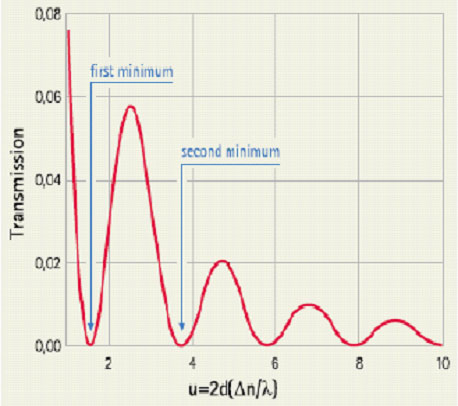﻿ Site MapTel: 440-232-8590
Fax: 440-232-8593
E-mail:

#### What is First Minima?

It is common for an LCD manufacturer to say they make "First Minima" LCD displays, but there is little information given as to exactly what "First Minima" is, or why you might need or want it. The concept is a bit tricky, but once you understand that there is just a simple formula that tells you how to build a first minima cell, the mystery goes away.

If you're a bit hazy on how an LCD is put together and how it operates, you may review our "Basic Operation of an LCD" page for a refresher.

Optimizing the Transmission of a 90o Twist Cell

The polarization state of light passing through a simple TN cell is a somewhat complex function first shown by Gooch and Tarry (1975). For a normal 90o twist TN cell, the transmission (T) between ideal polarizers is given as:

T = sin2 [F(1+u2)1/2]  / (1+u2)

where u=2d Dn / l

d = the cell gap of the display
F = twist angleof the cell (90o in a normal TN cell)
Dn = optical anisotropy of the display
l = wavelengthof the incident light

This equation for T is plotted in the graph shown below for a normal TN cell in its rest state,
where F = 90o. It shows that the transmission oscillates between maximum and minimum values, and will equal 0, that is perfectly linear polarized light will be 90o rotated, when:

u = 3½(First Minima), 15½(Second Minima), 35½(Third Minima).The cell gap (d) that will give minimum transmission for any cell in its rest state can be computed from the above formula using the Dn value provided by the LC fluid manufacturer. The first minima is the ideal place to build a cell, so u = 1.732 (3½) is the value we will use for u.

There is a wide range of values for modern LC fluids, but typical values for simple TN fluids range from about .85 to 1.2 or so.

An example calculation for a first minima cell using an LC fluid with a n value of .1154 and a desired wavelength of 550 nm would be:

u l / 2Dn = d

1.732 (550)  / 2(.1154) = d

4.13 mm = d

So if we build the display with a cell gap of 4.1 micron the display will have the maximum contrast that the technology will allow. Please note that the LC spacers used to make an LCD come in increments of .1 micron, so it is may be necessary to round off the results to the nearest tenth.

You may also have a question about what value to use for l. Our app note "Optimizing an LCD for Nighttime Viewing" might be usefull to determine this value. As usual, please give us a call at (440) 232-8590 if you have any questions about a custom LCD for your application.